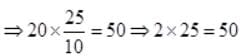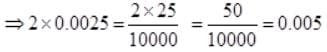Test: Operations on Large Numbers- 1

# Test: Operations on Large Numbers- 1

Test Description

## 10 Questions MCQ Test Mathematics for Class 5 | Test: Operations on Large Numbers- 1

Test: Operations on Large Numbers- 1 for Class 5 2023 is part of Mathematics for Class 5 preparation. The Test: Operations on Large Numbers- 1 questions and answers have been prepared according to the Class 5 exam syllabus.The Test: Operations on Large Numbers- 1 MCQs are made for Class 5 2023 Exam. Find important definitions, questions, notes, meanings, examples, exercises, MCQs and online tests for Test: Operations on Large Numbers- 1 below.
Solutions of Test: Operations on Large Numbers- 1 questions in English are available as part of our Mathematics for Class 5 for Class 5 & Test: Operations on Large Numbers- 1 solutions in Hindi for Mathematics for Class 5 course. Download more important topics, notes, lectures and mock test series for Class 5 Exam by signing up for free. Attempt Test: Operations on Large Numbers- 1 | 10 questions in 15 minutes | Mock test for Class 5 preparation | Free important questions MCQ to study Mathematics for Class 5 for Class 5 Exam | Download free PDF with solutions
 1 Crore+ students have signed up on EduRev. Have you?
Test: Operations on Large Numbers- 1 - Question 1

### Which of the following property of multiplication is shown in the statement given below? 3 x 6 = 6 x 3

Detailed Solution for Test: Operations on Large Numbers- 1 - Question 1

Commutative property: We can multiply in any order and get the same product.

Test: Operations on Large Numbers- 1 - Question 2

### The correct order to apply operations is

Detailed Solution for Test: Operations on Large Numbers- 1 - Question 2

According to BODMAS, the correct order of operations is Division - Multiplication - Addition - Subtraction.

Test: Operations on Large Numbers- 1 - Question 3

### 96 ÷ 2 = 48 is not equivalent to

Detailed Solution for Test: Operations on Large Numbers- 1 - Question 3

96 ÷ 2 = 48, Now evaluating the options, we get, 2 x 24 = 48, 4 x 12 = 48, 8 x 6 = 48 and 7 x 8 = 56.
Thus the solution other than 48 is the odd one out. Therefore is the odd one out.

Test: Operations on Large Numbers- 1 - Question 4

Which of the following equation shows the identity property of addition?

Detailed Solution for Test: Operations on Large Numbers- 1 - Question 4

Identity property: t = 0 + t, Adding zero does not change a number. Here 0 + 3 = 3 shows the identity property where adding zero does not change the sum.

Test: Operations on Large Numbers- 1 - Question 5

What should be subtracted from 14y to get 8 as answer?

Detailed Solution for Test: Operations on Large Numbers- 1 - Question 5

Let 'a' be subtracted from 14 y to get 8. Then, 14y - a = 8 ⇒ a = 14y - 8.

Test: Operations on Large Numbers- 1 - Question 6

If 4a + 3 = 15 then the value of 2a is

Detailed Solution for Test: Operations on Large Numbers- 1 - Question 6

4a + 3 = 15
⇒ 4a = 15 - 3 = 12
⇒ a = 12 ÷ 4 = 3
∴ 2a = 2 x 3 = 6

Test: Operations on Large Numbers- 1 - Question 7

86 - 23 = ? Which of the following statement is not equivalent to given statement?

Detailed Solution for Test: Operations on Large Numbers- 1 - Question 7

86 - 23 = 63, Now evaluating the options, we get 7 x 9 = 63, 3 x 21 = 63, 45 + 18 = 63 and 187 ÷ 2 = 62.333. Thus the solution other than 63 is the odd one out. Therefore is the odd one out.

Test: Operations on Large Numbers- 1 - Question 8

Which equation shows the commutative property of addition?

Detailed Solution for Test: Operations on Large Numbers- 1 - Question 8

Commutative property: r + s = s + r
We can add numbers in any order and get the same sum.
Here 8 + 4 = 4 + 8 shows the commutative property.

Test: Operations on Large Numbers- 1 - Question 9

Look at these numbers: 0.7, 0.5, 0.8, 0.7 Which two numbers in the box have a difference of 0.3?

Detailed Solution for Test: Operations on Large Numbers- 1 - Question 9

Look for numbers in the box that are greater than 0.3.

• Try 0.7. What number can you subtract from 0.7 to get 0.3? 0.7 - 0.4 = 0.3
0.4 is not in the box.
• Try 0.5. What number can you subtract from 0.5 to get 0.3? 0.5 - 0.2 = 0.3, 0.2 is not in the box.
• Try 0.8. What number can you subtract from 0.8 to get 0.3? 0.8 - 0.5 = 0.3
0.5 is in the box. The numbers 0.8 and 0.5 have a difference of 0.3.
Test: Operations on Large Numbers- 1 - Question 10

If  20 x 2.5 = 50 then 2 x 0.0025 = ?

Detailed Solution for Test: Operations on Large Numbers- 1 - Question 10

20 x 2.5 = 50## Mathematics for Class 5

12 videos|55 docs|35 tests
 Use Code STAYHOME200 and get INR 200 additional OFF Use Coupon Code
Information about Test: Operations on Large Numbers- 1 Page
In this test you can find the Exam questions for Test: Operations on Large Numbers- 1 solved & explained in the simplest way possible. Besides giving Questions and answers for Test: Operations on Large Numbers- 1, EduRev gives you an ample number of Online tests for practice

## Mathematics for Class 5

12 videos|55 docs|35 tests